Miscellaneous

Chapter 9 Class 11 Straight Lines
Serial order wise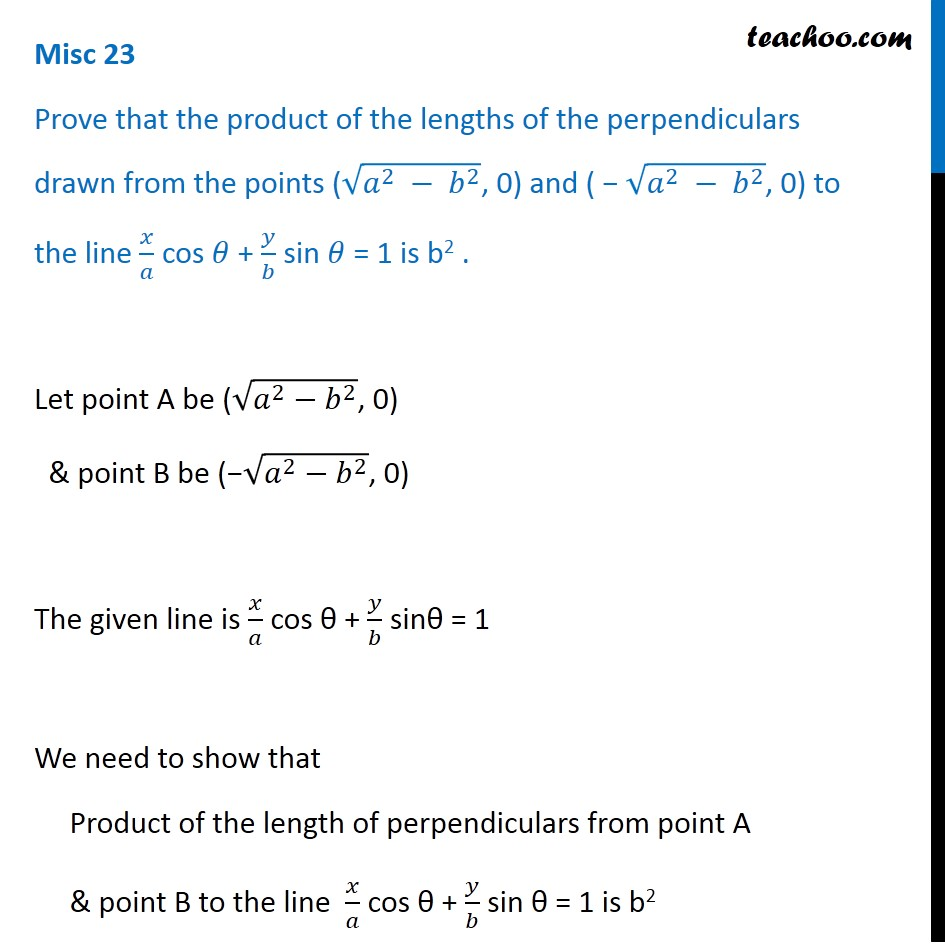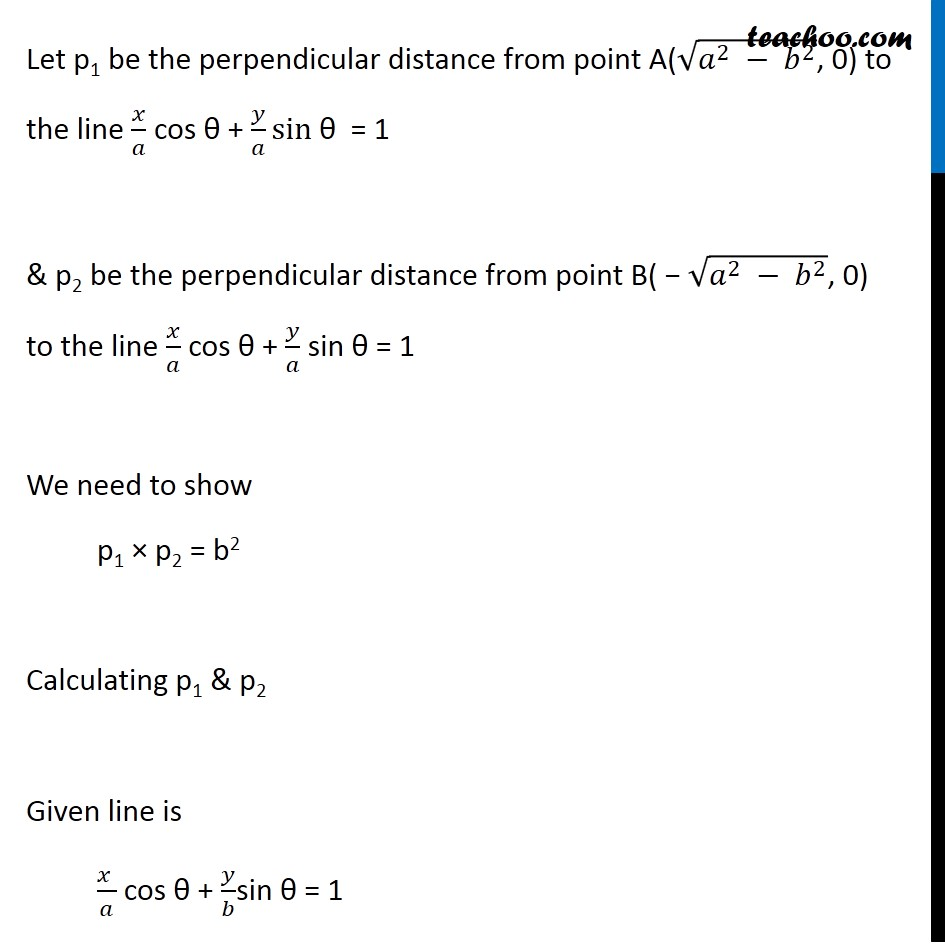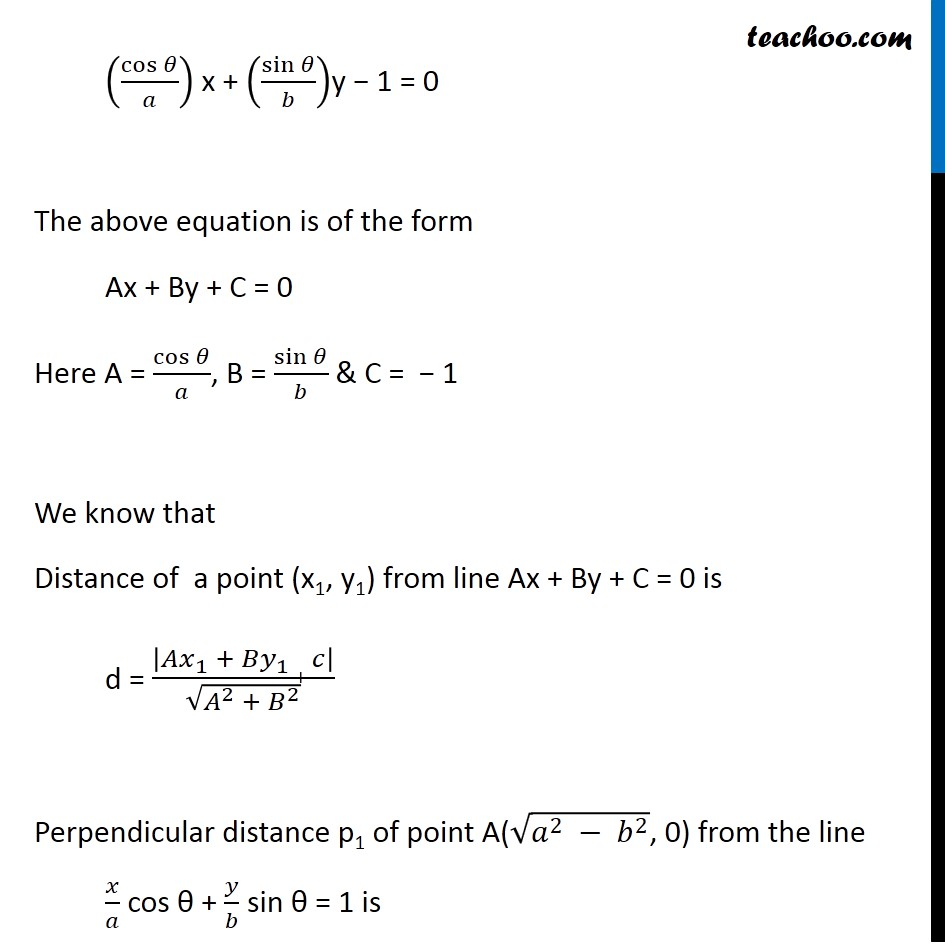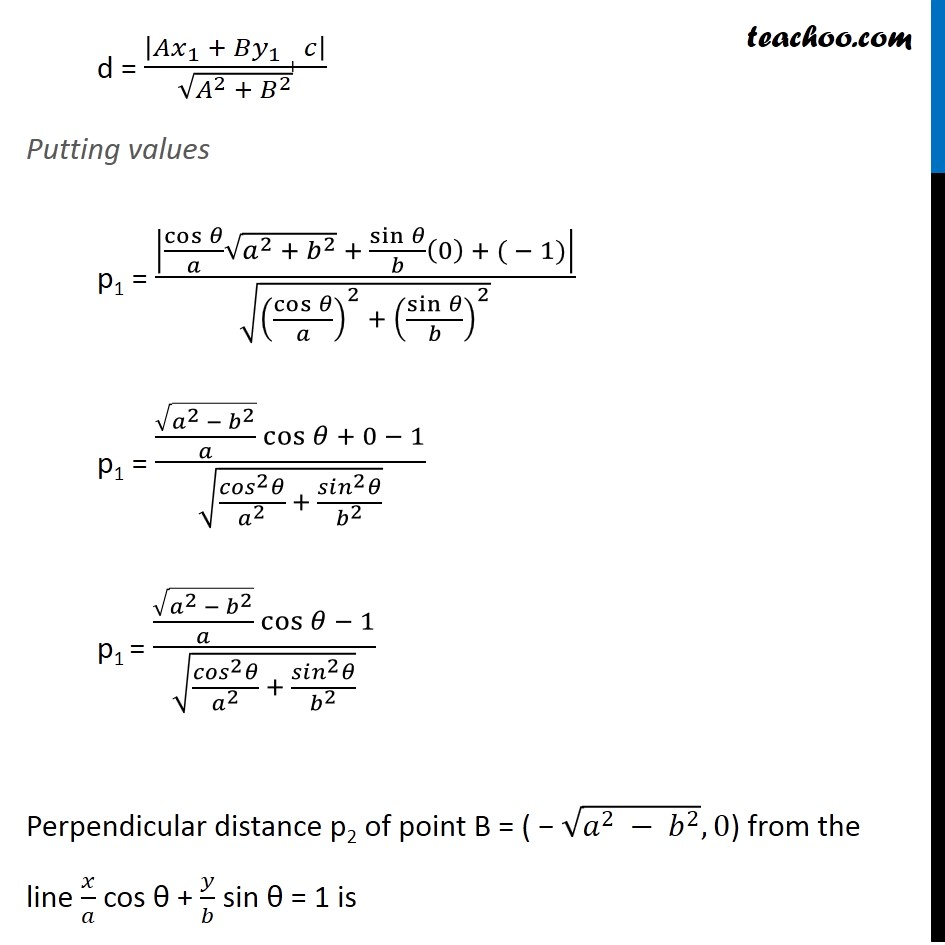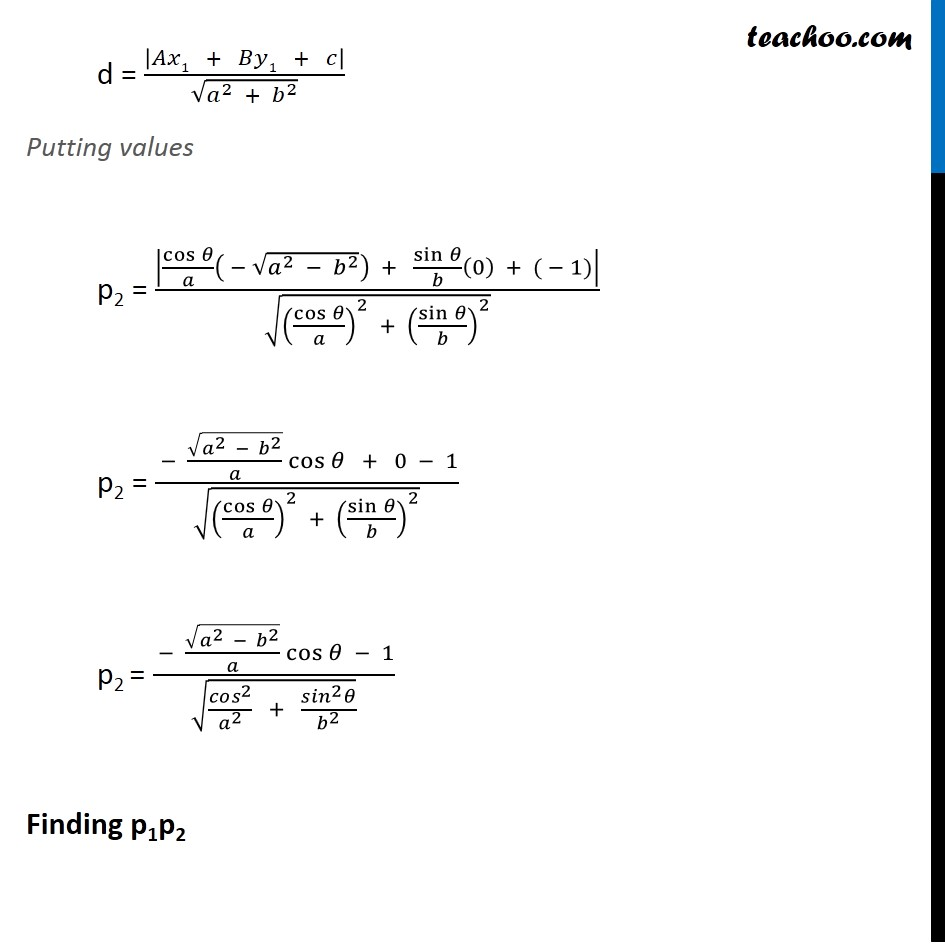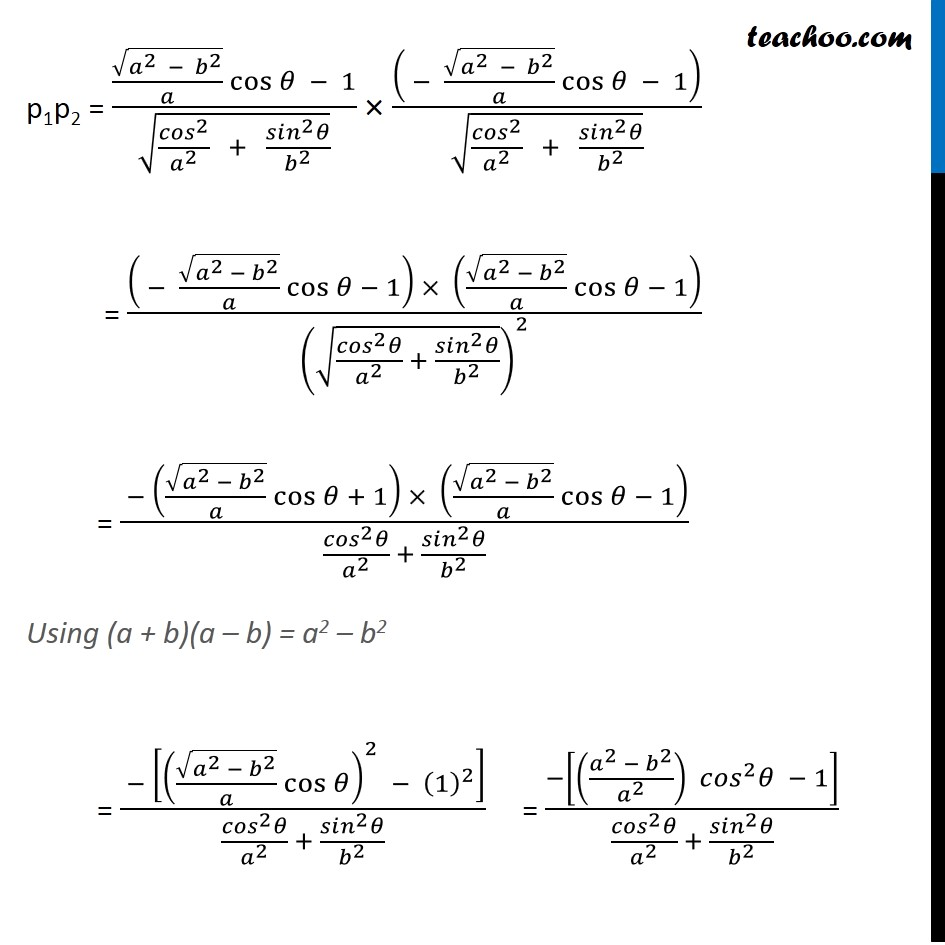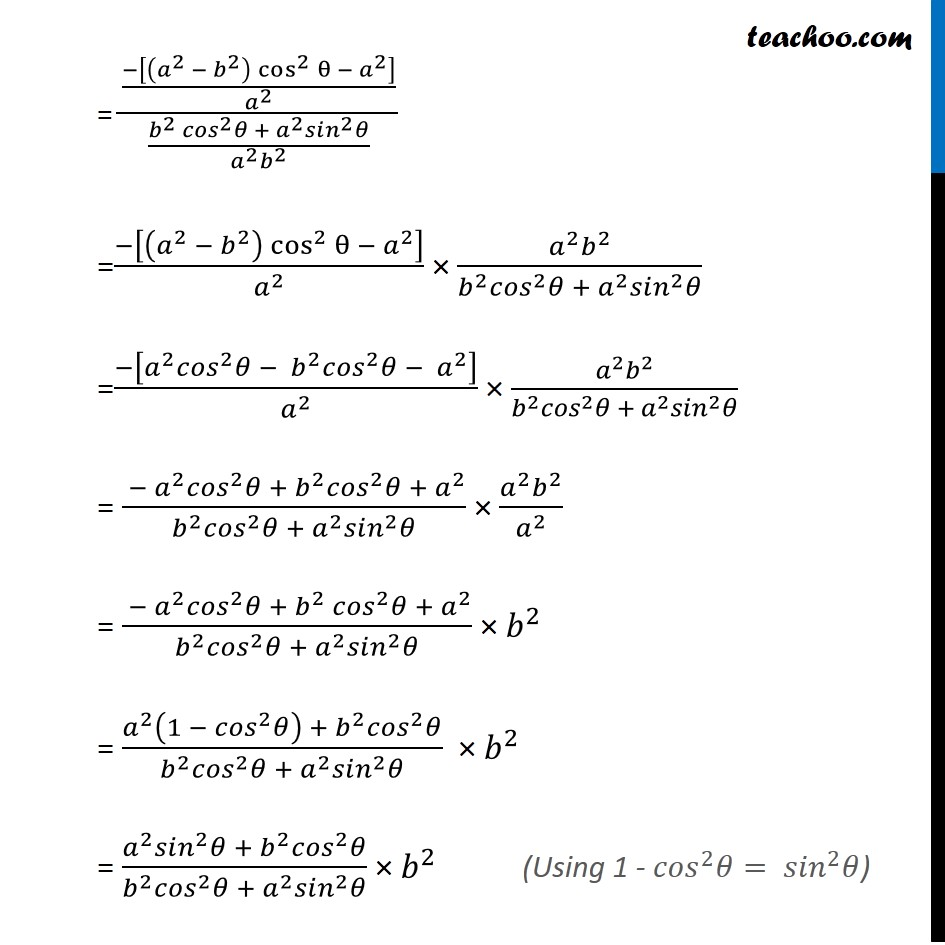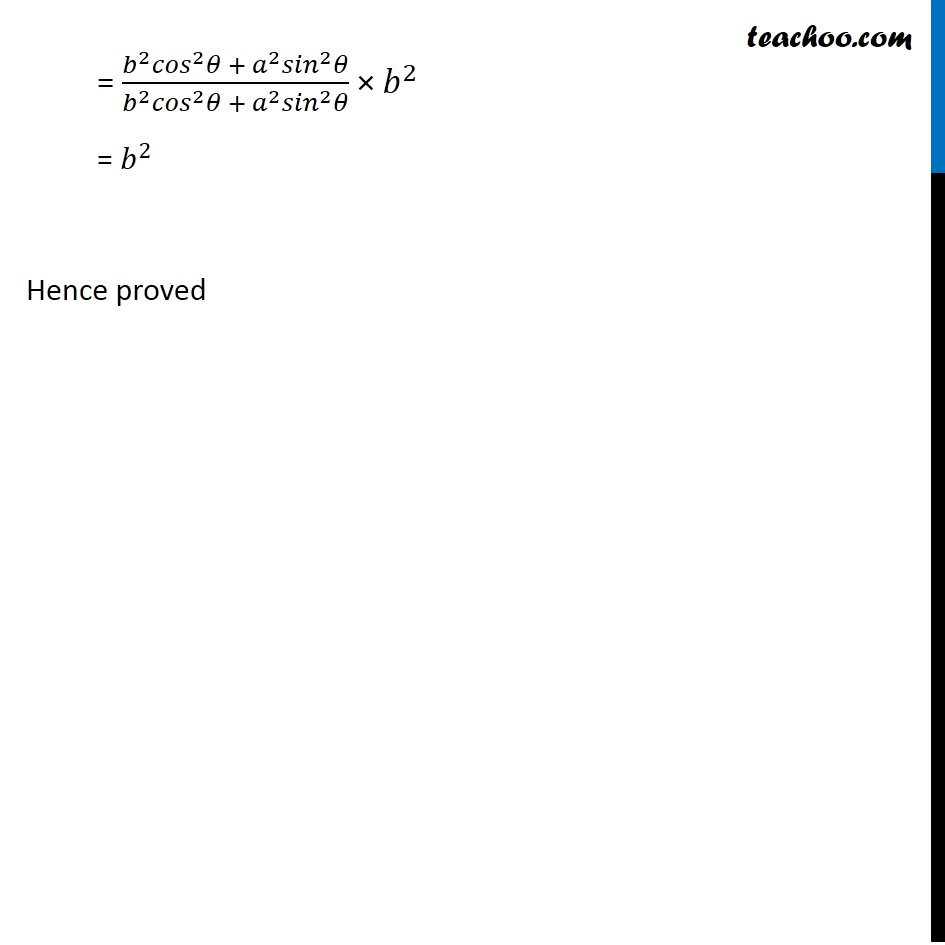Learn in your speed, with individual attention - Teachoo Maths 1-on-1 Class

### Transcript

Misc 22 Prove that the product of the lengths of the perpendiculars drawn from the points (√(𝑎^2 − 𝑏^2 ), 0) and ( − √(𝑎^2 − 𝑏^2 ), 0) to the line 𝑥/𝑎 cos 𝜃 + 𝑦/𝑏 sin 𝜃 = 1 is b2 . Let point A be (√(𝑎^2−𝑏^2 ), 0) & point B be (−√(𝑎^2−𝑏^2 ), 0) The given line is 𝑥/𝑎 cos θ + 𝑦/𝑏 sinθ = 1 We need to show that Product of the length of perpendiculars from point A & point B to the line 𝑥/𝑎 cos θ + 𝑦/𝑏 sin θ = 1 is b2 Let p1 be the perpendicular distance from point A(√(𝑎^2 − 𝑏^2 ), 0) to the line 𝑥/𝑎 cos θ + 𝑦/𝑎 sin θ = 1 & p2 be the perpendicular distance from point B( − √(𝑎^2 − 𝑏^2 ), 0) to the line 𝑥/𝑎 cos θ + 𝑦/𝑎 sin θ = 1 We need to show p1 × p2 = b2 Calculating p1 & p2 Given line is (𝑥 )/𝑎 cos θ + 𝑦/𝑏sin θ = 1 (cos⁡𝜃/𝑎) x + (sin⁡𝜃/𝑏)y − 1 = 0 The above equation is of the form Ax + By + C = 0 Here A = cos⁡𝜃/𝑎, B = sin⁡𝜃/𝑏 & C = − 1 We know that Distance of a point (x1, y1) from line Ax + By + C = 0 is d = |〖𝐴𝑥〗_1 + 〖𝐵𝑦〗_1 + 𝑐|/√(𝐴^2 + 𝐵^2 ) Perpendicular distance p1 of point A(√(𝑎^2 − 𝑏^2 ), 0) from the line 𝑥/𝑎 cos θ + 𝑦/𝑏 sin θ = 1 is d = |〖𝐴𝑥〗_1 + 〖𝐵𝑦〗_1 + 𝑐|/√(𝐴^2 + 𝐵^2 ) Putting values p1 = |cos⁡𝜃/𝑎 √(𝑎^2 + 𝑏^2 ) + sin⁡𝜃/𝑏 (0) + ( − 1)|/√((cos⁡𝜃/𝑎)^2 + (sin⁡𝜃/𝑏)^2 ) p1 = (√(𝑎^2 − 𝑏^2 )/𝑎 cos⁡𝜃 + 0 − 1)/√((〖𝑐𝑜𝑠〗^2 𝜃)/𝑎^2 + (〖𝑠𝑖𝑛〗^2 𝜃)/𝑏^2 ) p1 = (√(𝑎^2 − 𝑏^2 )/𝑎 cos⁡𝜃 − 1)/√((〖𝑐𝑜𝑠〗^2 𝜃)/𝑎^2 + (〖𝑠𝑖𝑛〗^2 𝜃)/𝑏^2 ) Perpendicular distance p2 of point B = ( − √(𝑎^2 − 𝑏^2 ), 0) from the line 𝑥/𝑎 cos θ + 𝑦/𝑏 sin θ = 1 is d = |𝐴𝑥1 + 𝐵𝑦1 + 𝑐|/√(𝑎^2 + 𝑏^2 ) Putting values p2 = |cos⁡𝜃/𝑎 ( − √(𝑎^2 − 𝑏^2 )) + sin⁡𝜃/𝑏 (0) + ( − 1)|/√((cos⁡𝜃/𝑎)^2 + (sin⁡𝜃/𝑏)^2 ) p2 = ( − √(𝑎^2 − 𝑏^2 )/𝑎 cos⁡𝜃 + 0 − 1)/√((cos⁡𝜃/𝑎)^2 + (sin⁡𝜃/𝑏)^2 ) p2 = ( − √(𝑎^2 − 𝑏^2 )/𝑎 cos⁡𝜃 − 1)/√(〖𝑐𝑜𝑠〗^2/𝑎^2 + (〖𝑠𝑖𝑛〗^2 𝜃)/𝑏^2 ) Finding p1p2